# Electrical Machines - Online Test

Q1. A 4 – point starter is used to start and control the speed of a
Explaination / Solution:
No Explaination.

Q2. A three-phase, salient pole synchronous motor is connected to an infinite bus. Ig is operated at no load a normal excitation. The field excitation of the motor is first reduced to zero and then increased in reverse direction gradually. Then the armature current
Explaination / Solution:
No Explaination.

Q3. A single phase air core transformer, fed from a rated sinusoidal supply, is operating at no load. The steady state magnetizing current drawn by the transformer from the supply will have the waveform
Explaination / Solution:

It is an air core transformer. So, there is no saturation effect.

Q4. A 220 V, DC shunt motor is operating at a speed of 1440 rpm. The armature resistance is 1.0 Ω and armature current is 10A. of the excitation of the machine is reduced by 10%, the extra resistance to be put in the armature circuit to maintain the same speed and torque will be
Explaination / Solution: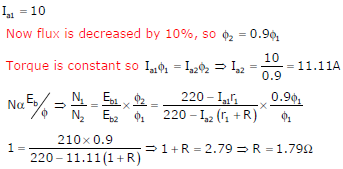Q5. A three –phase 440V, 6 pole, 50Hz, squirrel cage induction motor is running at a slip of 5%. The speed of stator magnetic field to rotor magnetic field and speed of rotor with respect to stator magnetic field are
Explaination / Solution: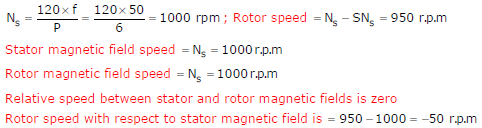Q6. The direct axis and quadrature axis reactance’s of a salient pole alternator are 1.2p.u and 1.0p.u respectively. The armature resistance is negligible. If this alternator is delivering rated kVA at upf and at rated voltage then its power angle is
Explaination / Solution: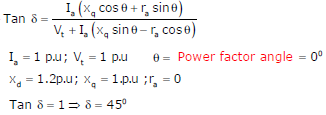Q7. A two-phase load draws the following phase currents: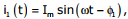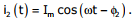These currents are balanced if ϕ1 is equal to
Explaination / Solution: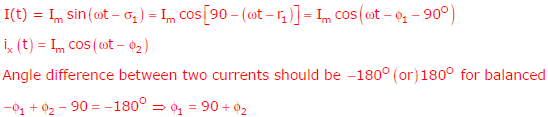Q8. The slip of an induction motor normally does not depend on
Explaination / Solution: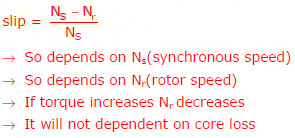Q9. A 220 V, 15 kW, 1000 rpm shunt motor with armature resistance of 0.25 Ω has a rated line current of 68 A and a rated field current of 2.2 A. The change in field flux required to obtain a speed of 1600 rpm while drawing a line current of 52.8 A and a field current of 1.8 A is
Explaination / Solution:Q10. The locked rotor current in a 3-phase, star connected 15 kW, 4-pole, 230 V, 50 Hz induction motor at rated conditions is 50 A. Neglecting losses and magnetizing current, the approximate locked rotor line current drawn when the motor is connected to a 236 V, 57 Hz supply is
Explaination / Solution: